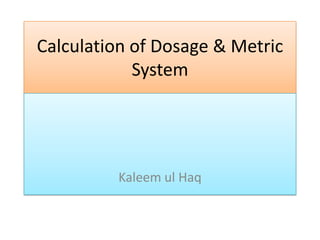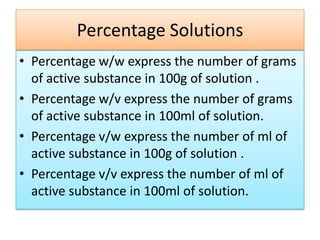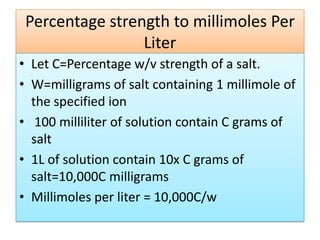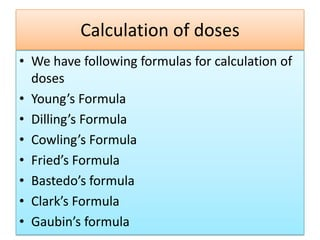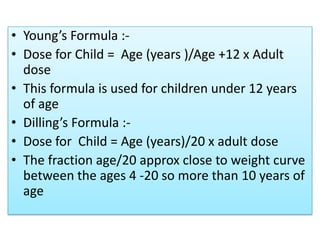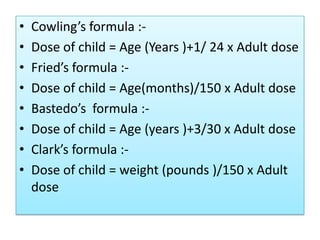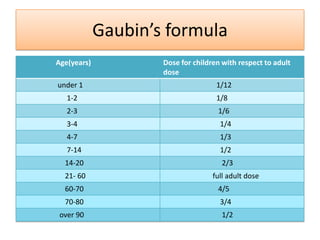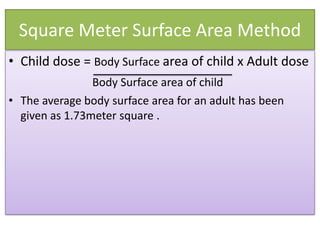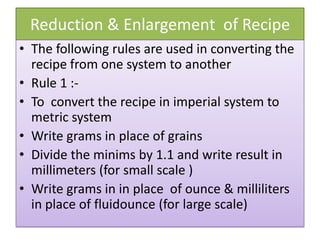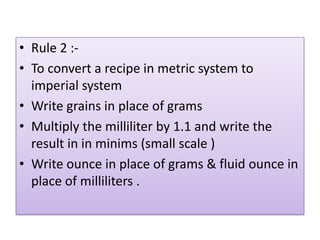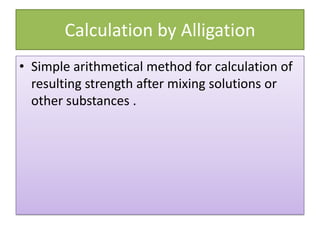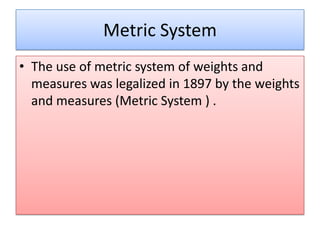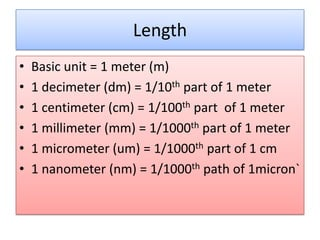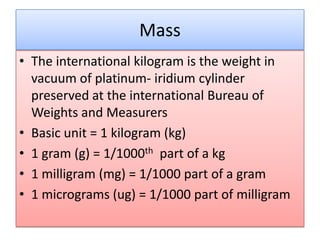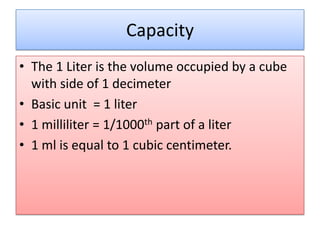1 of 15

### Calculation of dosage & metric system

1. Calculation of Dosage & Metric System Kaleem ul Haq
2. Percentage Solutions • Percentage w/w express the number of grams of active substance in 100g of solution . • Percentage w/v express the number of grams of active substance in 100ml of solution. • Percentage v/w express the number of ml of active substance in 100g of solution . • Percentage v/v express the number of ml of active substance in 100ml of solution.
3. Percentage strength to millimoles Per Liter • Let C=Percentage w/v strength of a salt. • W=milligrams of salt containing 1 millimole of the specified ion • 100 milliliter of solution contain C grams of salt • 1L of solution contain 10x C grams of salt=10,000C milligrams • Millimoles per liter = 10,000C/w
4. Calculation of doses • We have following formulas for calculation of doses • Young’s Formula • Dilling’s Formula • Cowling’s Formula • Fried’s Formula • Bastedo’s formula • Clark’s Formula • Gaubin’s formula
5. • Young’s Formula :• Dose for Child = Age (years )/Age +12 x Adult dose • This formula is used for children under 12 years of age • Dilling’s Formula :• Dose for Child = Age (years)/20 x adult dose • The fraction age/20 approx close to weight curve between the ages 4 -20 so more than 10 years of age
6. • • • • • • • • Cowling’s formula :Dose of child = Age (Years )+1/ 24 x Adult dose Fried’s formula :Dose of child = Age(months)/150 x Adult dose Bastedo’s formula :Dose of child = Age (years )+3/30 x Adult dose Clark’s formula :Dose of child = weight (pounds )/150 x Adult dose
7. Gaubin’s formula Age(years) Dose for children with respect to adult dose under 1 1/12 1-2 1/8 2-3 1/6 3-4 1/4 4-7 1/3 7-14 1/2 14-20 2/3 21- 60 full adult dose 60-70 4/5 70-80 3/4 over 90 1/2
8. Square Meter Surface Area Method • Child dose = Body Surface area of child x Adult dose Body Surface area of child • The average body surface area for an adult has been given as 1.73meter square .
9. Reduction & Enlargement of Recipe • The following rules are used in converting the recipe from one system to another • Rule 1 :• To convert the recipe in imperial system to metric system • Write grams in place of grains • Divide the minims by 1.1 and write result in millimeters (for small scale ) • Write grams in in place of ounce & milliliters in place of fluidounce (for large scale)
10. • Rule 2 :• To convert a recipe in metric system to imperial system • Write grains in place of grams • Multiply the milliliter by 1.1 and write the result in in minims (small scale ) • Write ounce in place of grams & fluid ounce in place of milliliters .
11. Calculation by Alligation • Simple arithmetical method for calculation of resulting strength after mixing solutions or other substances .
12. Metric System • The use of metric system of weights and measures was legalized in 1897 by the weights and measures (Metric System ) .
13. Length • • • • • • Basic unit = 1 meter (m) 1 decimeter (dm) = 1/10th part of 1 meter 1 centimeter (cm) = 1/100th part of 1 meter 1 millimeter (mm) = 1/1000th part of 1 meter 1 micrometer (um) = 1/1000th part of 1 cm 1 nanometer (nm) = 1/1000th path of 1micron`
14. Mass • The international kilogram is the weight in vacuum of platinum- iridium cylinder preserved at the international Bureau of Weights and Measurers • Basic unit = 1 kilogram (kg) • 1 gram (g) = 1/1000th part of a kg • 1 milligram (mg) = 1/1000 part of a gram • 1 micrograms (ug) = 1/1000 part of milligram
15. Capacity • The 1 Liter is the volume occupied by a cube with side of 1 decimeter • Basic unit = 1 liter • 1 milliliter = 1/1000th part of a liter • 1 ml is equal to 1 cubic centimeter.